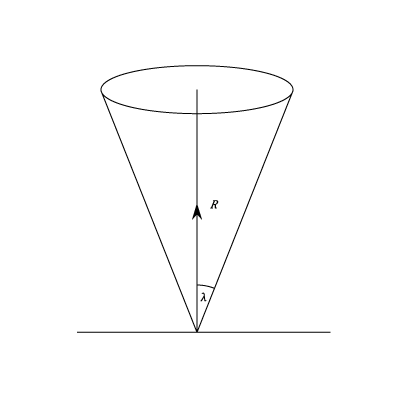I have forgotten

•http://facebook.com/
•https://www.google.com/accounts/o8/id
•https://me.yahoo.com

# Angle and Cone of friction

The relationship between the Angle and Cone of FrictionView other versions (4)

## Description

Engineers often refer to either the coefficient of friction or the angle of friction. This section describes their relationship and goes on to explain the Cone of friction:

Let R be the normal reaction at a point of contact O and F the frictional force acting in a direction perpendicular to R. Then the total force at O is given byacting in a direction making an anglewith the normal reaction.

If friction is limiting,and the action at O makes an angle ofwith the normal reaction. This angle is denoted by.

Thusand the magnitude of the limiting friction can be found if eitheroris known.

If the direction in which the body tends to move is varied the the force of limiting friction will always lie in the plane through O perpendicular to the normal reaction and the direction of the total action at O will always lie 0n a cone with it's vertex at O and axis along the line of the normal reaction. The semi vertical angle will beThis cone is called the cone of friction. If friction is not limiting, the angle made by the total action at O will be less than. Hence whether the friction be limiting or not, the direction of the total action at O must be inside or on the cone of friction. It follows that if P is the resultant of the other forces acting on the body which is in equilibrium, the direction of P must lie inside or on the cone o friction, since P must balance the total action at O